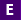#Not a Florida public school educator?

Access this resource
on CPALMS.com

### General Information

Subject(s): Mathematics
Grade Level(s): 9, 10, 11, 12
Intended Audience: Educators, Students
Suggested Technology: Computers for Students, Internet Connection
Instructional Time: 30 Minute(s)
Keywords: linear inequalities, interval notation, graph linear inequality
Instructional Component Type(s):
Resource Collection: General Collection

## Aligned Standards

This vetted resource aligns to concepts or skills in these benchmarks.

## Related Resources

Other vetted resources related to this resource.

## Tutorial

Linear Equations in One Variable:

This lesson introduces students to linear equations in one variable, shows how to solve them using addition, subtraction, multiplication, and division properties of equalities, and allows students to determine if a value is a solution, if there are infinitely many solutions, or no solution at all. The site contains an explanation of equations and linear equations, how to solve equations in general, and a strategy for solving linear equations. The lesson also explains contradiction (an equation with no solution) and identity (an equation with infinite solutions). There are five practice problems at the end for students to test their knowledge with links to answers and explanations of how those answers were found. Additional resources are also referenced.

Type: Tutorial